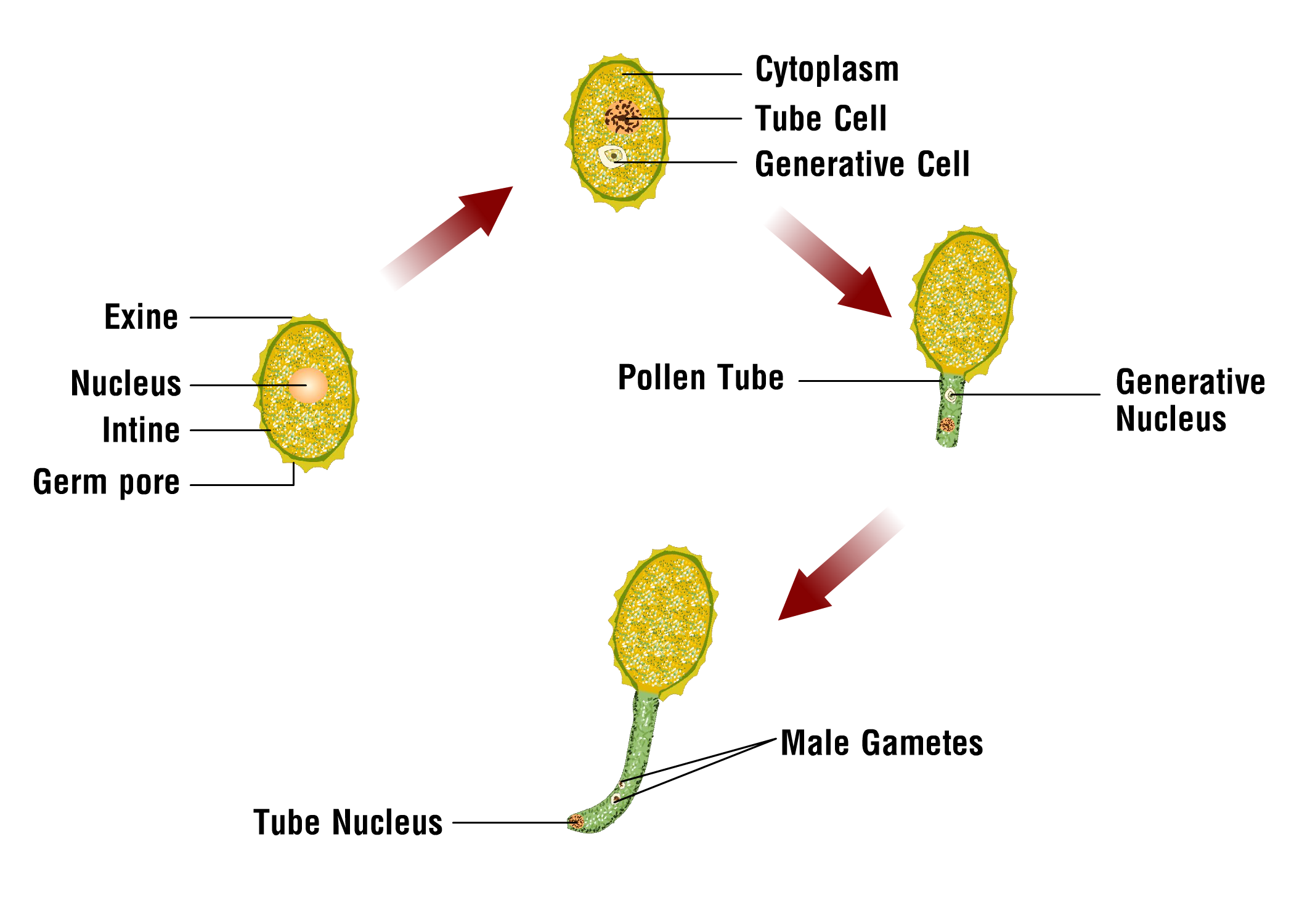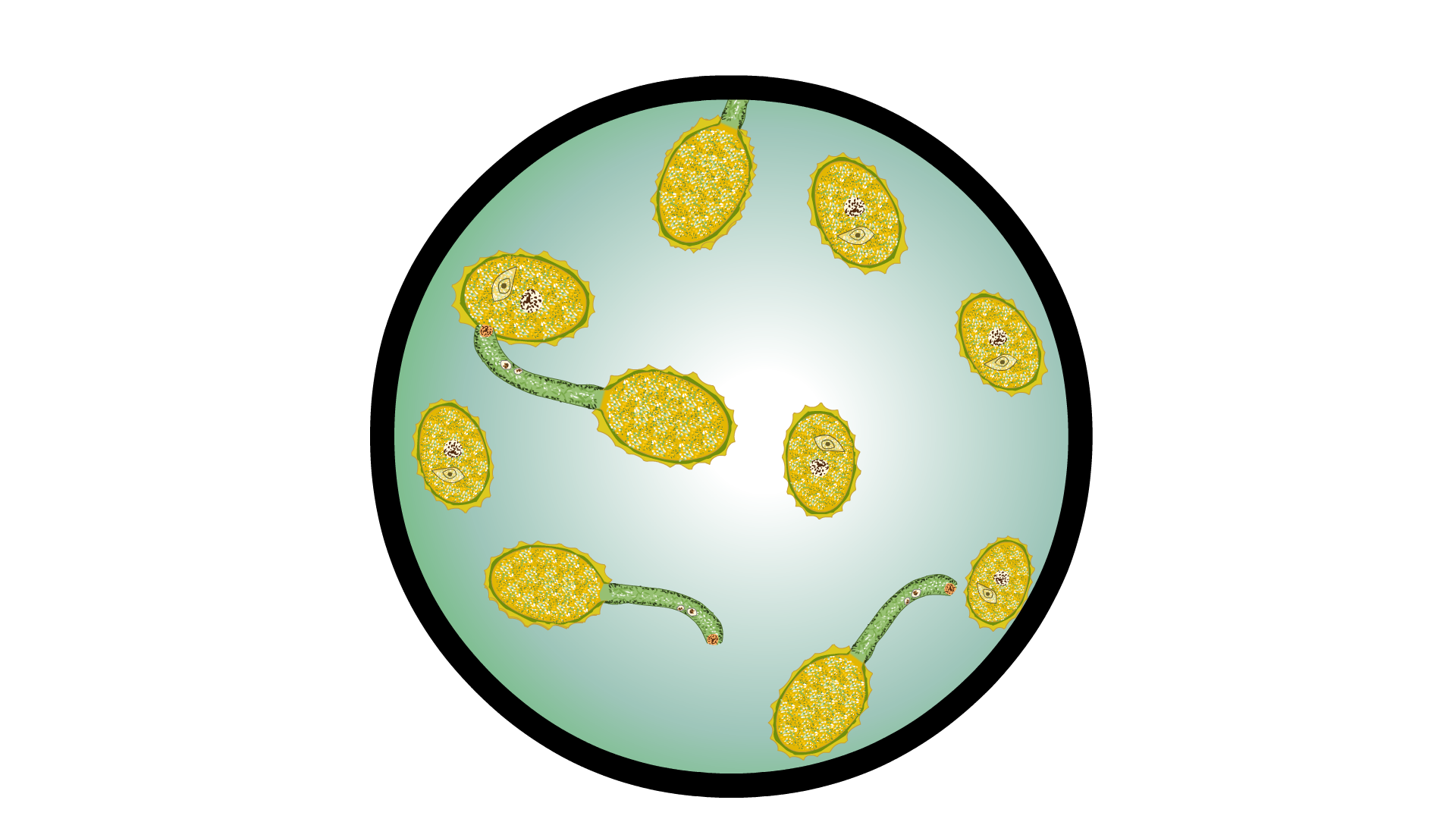Percentage of Pollen Germination

# Our objective

To calculate percentage of pollen germination

# The Theory

In nature, pollen grains germinate on the compatible stigmas of the carpel. The pollen grain reaches the stigma to begin the process of pollen germination. The germination of pollen grains on the stigma begins with the absorption of water and nutrients. The pollen grain's cell divides into two cells: the tube cell, which is larger, and the generative cell, which is smaller in size. Intine is the inner layer of pollen that extends out through the germ pore to generate the pollen tube. By this time, the generative nucleus divides into two male gametes and the tube nucleus degenerates (see figure 1).

Male gametes are produced when the pollen tube bursts, and one of them fuses with an egg cell and the other two with two polar nuclei. The pollen tube in the flower then develops in the direction of the ovule, where it releases the sperm, produced in the pollen grain for fertilization. The adult male microgametophyte of these plants is the germinated pollen grain with its two sperm cells.Figure1: Pollen germination

## How to calculate pollen germination?

• The percentage of pollen germination can be calculated by the formula, (n/N) X 100
• ‘n’ is the total number of germinated pollen grains in a field of microscope and
• ‘N’ is the total number of pollen grains in a field of microscope.

The image below shows the microscopic view of pollen germination.Figure 2: Pollen germinates in microscopic field

 Number of Pollen grains germinated (n) Total number of pollen grains (N) Percentage of pollen grains germinated [(n/N)*100] 5 50 (5/10)*100 = 10% 10 50 (10/50)*100 = 20%

# Learning Outcomes

• Students understand the idea of pollen germination occurs in nature
• Students become familiar with the idea of pollen germination in a nutrient medium.
• Students will learn how to calculate the percentage of pollen germination.